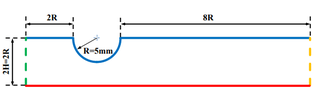# Pressure drop in channel

• Darcy30

#### Darcy30

Hallo. Since I'm new in this field, I hope someone can help me.
I have a laminar, steady state, incompressible flow in a channel (a fully developed).
Geometry of the channel is on the photo.
How should I calculate the drop of static pressure across the channel?
Since I have Re, I did calculate a inlet velocity, but now I'm stuck.
Thanks

#### Attachments

•channel.png
2.7 KB · Views: 102
•FluidDroog

To do this, you need to solve the Naiver Stokes equations, probably numerically. To get a lower bound to the pressure drop, you can at least solve analytically for the case where you remove the constriction and have rectilinear flow between parallel plates.

•FluidDroog
Hi Darcy30,

I understand the problem as follows:
• Assume laminar, steady state, constant density (incompressible), fully developed flow.
• Channel Geometry and Fluid Properties are known (I will assume the channel is circular)
• The inlet velocity is known
Goal:
• Find the pressure drop across a channel.
Solution Hint:
Darcy Friction Factor, Moody Diagram

Solution:

The pressure drop down a pipe is related to the friction factor.
For Laminar flow,
$$f_D = \frac{64}{Re}$$
If not, then you will need to assume smooth walls or know the surface roughness.

The pressure drop is related to the following.
$$dP = f_D * \frac{\rho*V^2*L}{2*D}$$
Break the problem into 3 sections. Inlet straight pipe, the circular restriction, and the outlet straight pipe. The inlet and outlet is straight forward. You will add up the dP for each section to find your answer.

The tricky part of this problem is that you need to integrate the friction factor (hence pressure drop) over the circular restriction. You need a formula for the relationship of Pipe Diameter, D, to length across the channel, x. Something like this:
$$Dtube = 2 * R - R*sin(acos(R/x))$$
Additionally, the velocity changes based on cross-sectional area. Use the conservation of mass to find that
$$mdot = \rho*Area*Velocity$$
There is symmetry, so we only need to consider a 1/4 circle and multiply the friction factor by 2. Integrate from x = 0 to R. Or solve it numerically in a spreadsheet program with small steps of x.

PS: If the channel is indeed non-circular, then use the hydraulic diameter Dh in place of D.
$$Dh = \frac {4*Area}{Perimeter}$$

References:
 Moody Diagram https://en.wikipedia.org/wiki/Moody_chart?msclkid=7b62d23aaee911ec823e165de974644a
 Hydraulic Diameter https://en.wikipedia.org/wiki/Hydraulic_diameter?msclkid=0899a52baef711ec890e442c37cca7b6

What is the exact statement of the problem?